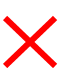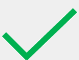# 4.23: Balancing Chemical Equations: Determining Balancing Coefficients

$$\newcommand{\vecs}{\overset { \rightharpoonup} {\mathbf{#1}} }$$ $$\newcommand{\vecd}{\overset{-\!-\!\rightharpoonup}{\vphantom{a}\smash {#1}}}$$$$\newcommand{\id}{\mathrm{id}}$$ $$\newcommand{\Span}{\mathrm{span}}$$ $$\newcommand{\kernel}{\mathrm{null}\,}$$ $$\newcommand{\range}{\mathrm{range}\,}$$ $$\newcommand{\RealPart}{\mathrm{Re}}$$ $$\newcommand{\ImaginaryPart}{\mathrm{Im}}$$ $$\newcommand{\Argument}{\mathrm{Arg}}$$ $$\newcommand{\norm}{\| #1 \|}$$ $$\newcommand{\inner}{\langle #1, #2 \rangle}$$ $$\newcommand{\Span}{\mathrm{span}}$$ $$\newcommand{\id}{\mathrm{id}}$$ $$\newcommand{\Span}{\mathrm{span}}$$ $$\newcommand{\kernel}{\mathrm{null}\,}$$ $$\newcommand{\range}{\mathrm{range}\,}$$ $$\newcommand{\RealPart}{\mathrm{Re}}$$ $$\newcommand{\ImaginaryPart}{\mathrm{Im}}$$ $$\newcommand{\Argument}{\mathrm{Arg}}$$ $$\newcommand{\norm}{\| #1 \|}$$ $$\newcommand{\inner}{\langle #1, #2 \rangle}$$ $$\newcommand{\Span}{\mathrm{span}}$$$$\newcommand{\AA}{\unicode[.8,0]{x212B}}$$

Learning Objectives
• Use coefficients to balance a chemical equation.

The previous section of this chapter presented and discussed a chemical equation that was balanced, as written, and, therefore, did not require the incorporation of any coefficients.  However, the inclusion of one or more balancing coefficients is typically necessary for most chemical equations.

For example, consider the following chemical equation.  In order to balance this equation, coefficients should be written in the "blanks," as necessary.

___ $$\ce{F_2} \left( g \right) +$$ ___ $$\ce{LiBr} \left( aq \right) \rightarrow$$ ___ $$\ce{LiF} \left( aq \right) +$$ ___ $$\ce{Br_2} \left( l \right)$$

In order to balance a chemical equation, the quantities of each type of element and polyatomic ion that are present in the reactants and the products of the reaction must be determined.  No polyatomic ions are present in any of the formulas that are shown above.  Therefore, only individual elements must be considered for this reaction.

The quantities of fluorine, F, lithium, Li, and bromine, Br, that are present in the given equation are summarized in the table that is shown below.

Element or Ion Reactants Products Balanced
F 2 1Li 1 1Br 1 2In order for a component of a reaction to be balanced, the reactants and products of the reaction must contain identical total quantities of that type of element or polyatomic ion.  Since both sides of the reaction contain equal amounts of lithium, Li, this element is balanced.  However, fluorine, F, and bromine, Br, are not balanced, because each of these elements is present in different quantities on the reactant and product sides of the reaction arrow.  Therefore, one or more coefficients must be written in the "blanks" above, in order to balance this reaction.

When determining the value of a coefficient, do not

• attempt to balance multiple different elements or polyatomic ions with a single coefficient,
• begin the balancing process by selecting an element or a polyatomic ion that is present in multiple formulas on the same side of the reaction arrow, or
• start by balancing a chemical that is represented in its atomic form.

Once an acceptable component has been selected,

• divide the larger quantity of that chemical by the corresponding smaller count,
• write the number that results in the coefficient "blank" on the side of the reaction arrow that has fewer of that component, and
• multiply the subscript of each element or polyatomic ion that present in the corresponding chemical formula by the value of the coefficient that was incorporated, in order to update the quantities of those components.

The process described above should be repeated until all of the elements and polyatomic ions that are present in the reaction are balanced.

Neither of the unbalanced components in this reaction is present in multiple formulas on the same side of the reaction arrow or is represented in its atomic form.  Therefore, the balancing process could be initiated by selecting either fluorine, F, or bromine, Br, for further analysis.

In order to balance fluorine, F, a coefficient should be written in the "blank" that corresponds to lithium fluoride, LiF, on the right side of the equation, as fewer fluorines are present on this side of the reaction arrow.  The value of this coefficient, 2, is determined by dividing the larger quantity of this element, 2, by its smaller count, 1.  Inserting this coefficient results in the chemical equation that is shown below.

___ $$\ce{F_2} \left( g \right) +$$ ___ $$\ce{LiBr} \left( aq \right) \rightarrow$$ 2 $$\ce{LiF} \left( aq \right) +$$ ___ $$\ce{Br_2} \left( l \right)$$

As lithium fluoride, LiF, contains both lithium, Li, and fluorine, F, incorporating this coefficient alters the amounts of these elements on the product side of the equation.   The updated quantities of these elements, which are obtained by multiplying the subscripts that are associated with each elemental symbol by the coefficient that was written, are reflected in the table that is shown below.  Inserting this coefficient balances fluorine, F, as intended.  However, the quantities in which lithium, Li, are present, which had been identical, are now unequal.  Therefore, this element must now be balanced, as well.

Element or Ion Reactants Products Balanced
F 2 $$\cancel{\rm{1} }$$ 2Li 1 $$\cancel{\rm{1} }$$ 2Br 1 2In order to balance bromine, Br, a coefficient should be written in the "blank" that corresponds to lithium bromide, LiBr, on the left side of the equation, as fewer bromines are present on this side of the reaction arrow.  The value of this coefficient, 2, is determined by dividing the larger quantity of this element, 2, by its smaller count, 1.  Inserting this coefficient results in the chemical equation that is shown below.

___ $$\ce{F_2} \left( g \right) +$$ 2 $$\ce{LiBr} \left( aq \right) \rightarrow$$ 2 $$\ce{LiF} \left( aq \right) +$$ ___ $$\ce{Br_2} \left( l \right)$$

As lithium bromide, LiBr, contains both lithium, Li, and bromine, Br, incorporating this coefficient alters the amounts of these elements on the reactant side of the equation.  The updated quantities of these elements, which are obtained by multiplying the subscripts that are associated with each elemental symbol by the coefficient that was written, are reflected in the table that is shown below.  Inserting this coefficient balances bromine, Br, as intended.  Additionally, the quantities in which lithium, Li, are present, which had been unequal, are now identical.  Therefore, this element is now balanced, as well.

Element or Ion Reactants Products Balanced
F 2 $$\cancel{\rm{1} }$$ 2Li $$\bcancel{\rm{1} }$$ 2 $$\cancel{\rm{1} }$$ 2Br $$\bcancel{\rm{1} }$$ 2 2After balancing all of the chemicals that are present in a reaction, confirm that all coefficients are whole numbers.  If any fractional coefficients were used to balance an equation, all of the coefficients in that equation must be multiplied by an appropriate value, in order to cancel the fractional value.  If possible, the coefficients must be reduced to the lowest-common ratio of whole numbers by dividing all of the coefficients by the same numerical value.  However, this division should only be performed if all of the resultant coefficients remain whole numbers.  Finally, as indicated previously, values of "1" are usually implicitly-understood in chemistry and should not be written the coefficient "blanks" in a chemical equation.

No fractional coefficients are written in the equation that is shown above.  Furthermore, because of the unwritten "1"s that are understood to occupy the first and last "blanks" in this equation, these coefficients cannot be divided.  Therefore, the final equation that is presented above is chemically-correct, as written.

4.23: Balancing Chemical Equations: Determining Balancing Coefficients is shared under a not declared license and was authored, remixed, and/or curated by LibreTexts.